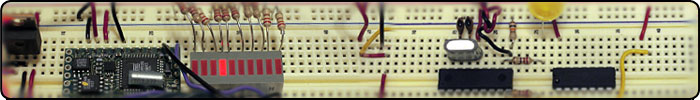Analog Input (BX-24): Input to the BX-24 can be in two forms. Analog or digital. Analog is most intuitive because this is the kind of input we get from our senses such as sound, light, touch etc. Analog is typically described as a continuous set of values instead of a single on/off response (digital). In this example I have wired up a 5kOhm potentiometer (pot) to pin 13 of the BX-24. Note that the black wire from the pot goes to ground, the yellow wire goes to 5VDC, and the red wire goes to pin 13 of the BX-24. As the knob on the potentiometer rotates we get a change in voltage. The multimeter shows the that 2.12 volts are going into pin 13 of the BX-24.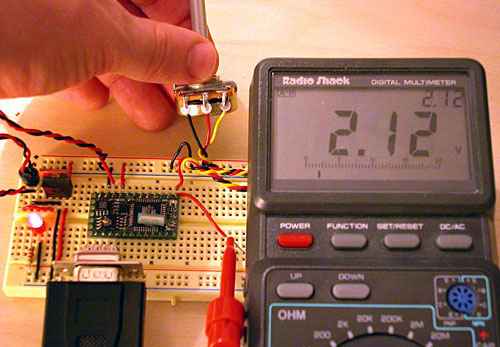How it works: While the voltage going into pin 13 can range from 0-5 volts, the BX-24 converts the continous voltage levels into digital values using an analog to digital converter (ADC). Pin 13 - 20 can accept analog values and convert them to 10-bit numbers. For a 10-bit number the values can range from 0 to 1024. I've pasted the code below to display the digital output that corresponds to the analog voltage input. ```dim potVar as integer sub main() call delay(0.5) ' start program with a half-second delay do potVar = getADC(13) debug.print "potVar = " ; cstr(potVar) loop end sub ``` LED bargraph: The next figure shows how to use the same 5kOhm potentiometer with an LED bargraph. First we get input from the potentiometer via pin 19. This gives us values on the range from 0 to 1024. I've wired the LED bargraph so that pin 5 illuminates LED 1, pin 6 illuminates LED 2, and so on.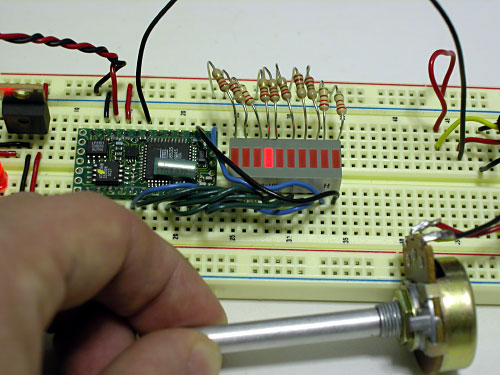How it works: As you rotate the knob on the potentiometer, one of the LED bars is illuminated. As you turn the knob to the left the illuminated LED moves to the left (same for right). The trick here is to convert potVar = getADC(19) into a corresponding pin number. First, because the range of numbers is 0 to 1024, I want to rescale the values so that I get 1 to 10, which is the number of LEDs on the bargraph. To do this I figured out how many steps I needed by dividing 1024 by 9 (which equals 113.78). I divided potVar by 113, and then added an offset of 5 to account for the pin numbering convention for the BX-24. call pulseOut(pinNum,10000,1) requires that pinNum be a byte value. This is why I caste the integer result as a byte (also because whenever you use getADC it returns a 10bit integer, and I really only needed values in the range of 5 to 14). All this was accomplished in the line of code: pinNum = cbyte((potVar\113) + 5). Another neat exercise would be to display the integer value on the LED bargraph as a binary number. Given that there are 10 LEDs and that potentiometer value is a 10bit number, this should be pretty simple. I'll save that for another day. ```dim potVar as integer dim pinNum as byte sub main() call putPin(14,1)'briefly check the status of the LED bargraph call delay(0.5) ' with a half-second delay on LED # 10 call putPin(14,0) do potVar = getADC(19) 'debug.print "potVar = " ; cstr(potVar) pinNum = cbyte((potVar\113) + 5) 'divide by 113 so you get values in the range from 1 to 10 'add five to account for pin location 'cast the final value as a byte debug.print "pinNum = " ; cstr(pinNum) 'pulse the pin number high for 10000 microseconds call pulseOut(pinNum,10000,1) loop end sub ```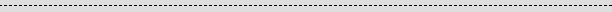<-- back to microcontrollers/breadboards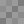Copyright © 2006 Jason Babcock. All rights reserved. Valid CSS   Valid XHTML 1.0